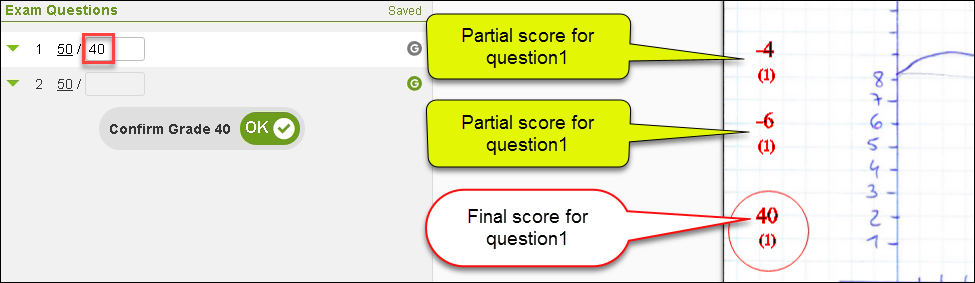Also available in a video... CLICK HERE to watch the video tutorial

What is a partial score for a question?A partial score makes it possible to subtract or add points to the same question continuously;

If, for example, we want to grade a question and subtract from its maximum score several times during the exam grading.

How to use the Minus and Plus buttons?

Choose the plus or minus button and click on the notebook and the "Grade Question" window will open accordingly.
Enter the number of points to subtract or to addWe can predefine the negative or positive score by selecting it from the "points menu" so that it is entered automatically in the "Grade Question" window

(the range is limited to a positive / negative score of up to 10 points)A positive or negative score may be given several times to the question.
The score will be calculated and automatically updated.
To complete grading the question, we will use one of the "final grade" buttons (G or mini G ) to give a final score,
we will not need to enter the score at the "Grade Question" window as it will be calculated automaticallyImportant notes:
• Negative and positive scores can not be used together for the same question
• The option is available only when Exam Structure is set for the exam
• After entering the partial score it is not necessary to give a final score to the question (with the 'G' button), however it is recommended so it will be updated as a final score , and also so that the student can see the final score on the exam